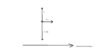# Box on a conveyor belt (friction)

• sp3sp2sp

## Homework Statement

A 10kg crate is placed on a horizontal conveyor belt. Draw a free-body diagram showing all the forces on the crate if the conveyer belt is speeding up.

## Homework Equations

Its mastering physics says to "Draw the force vectors with their tails at the dot. The orientation of your vectors will be graded. The exact length of your vectors will not be graded but the relative length of one to the other will be graded. "
The only other thing is there is long arrow pointing to right labelled "direction".

## The Attempt at a Solution

I know that normal force will go perp to surface so positive y-axis, and F_mg will go along negative x-axis, but the friction force is making me think...The static friction force will point opposite the direction the object would move if there was no friction. The crate would move to left in this scenario, because it is on a conveyor belt accelerating to right. But that's what confuses me, because that would actually be the same direction as conveyor belt. Is this because of 2 reference frames, or nothing to do with that? thanks for any help.

I know that normal force will go perp to surface so positive y-axis, and F_mg will go along negative x-axis,
The mg force of gravity is down, not along the x axis...

And the horizontal force of friction is what is moving the box sideways and accelerating it.

Can you use the Upload button in the lower right of the Edit window to Upload a PDF or JPEG image of your FBD? Thanks.

sorry i actually did mean to say mg pointing along neg-y axis. FBD attached.

#### Attachments

•conveyor problem.jpg
6.4 KB · Views: 1,649
if the box was on a frictionless surface it would move to left if conveyor belt moved to right. So that's why I am saying static friction points to right, to oppose motion it would move.

if the box was on a frictionless surface it would move to left if conveyor belt moved to right. So that's why I am saying static friction points to right, to oppose motion it would move.
I agree with what you are saying, but the wording could be better.

"If the box was on a frictionless surface it would move left relative to a rightward-accelerating belt".
"Static friction acts rightward on the box, opposing the relative motion that would otherwise exist".

On your drawing, the vector denoting the force of gravity is drawn longer than the vector denoting the normal force. Is that intentional?

no i just drew it quick. Downward force of gravity, g must = normal force, N because no movement in y directions.
... if my fbd is correct then that means that that friction points opposite to the direction of motion only with respect to nonrelative motion, is that right? thanks

if my fbd is correct then that means that that friction points opposite to the direction of motion only with respect to nonrelative motion, is that right?
I do not understand what you are trying to say.

Static friction acts on an object to oppose the relative motion that would exist were it not for static friction. "nonrelative motion" (whatever that means) is irrelevant. Dynamic friction acts to oppose the relative motion that actually does exist. "nonrelative motion" (whatever that means) is irrelevant.

The relevant relative motion is between the two objects that are in contact.